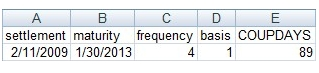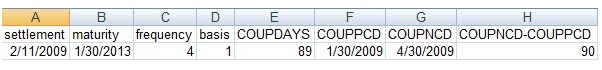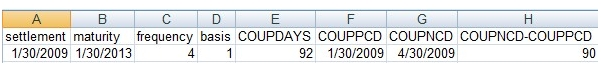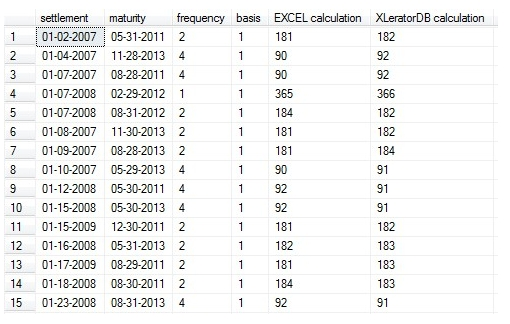## COUPDAYS difference between XLeratorDB and EXCEL

Jun 30

Written by: Charles Flock
6/30/2009 6:08 PMA brief explanation on why the XLeratorDB implementation of this function is different than the EXCEL implementation.

The COUPDAYS function calculates the number of days in the coupon period. The inputs into this function are the settlement date, maturity date, frequency of the interest payments, and the basis. For the most part, the calculation is fairly straight forward; you simply take the assumed number of days in the year associated with the basis code and divide it by the frequency.
The Actual/Actual basis code works differently, however. It calculates the actual number of days in the coupon period. The coupon period is derived from the settlement date and the maturity date passed into the function. Coupon dates are calculated backwards from the maturity date, using the frequency. In the bond markets, the convention is that the period that the settlement date falls in is the period where the settlement date is greater than or equal to the previous coupon date and less than the next coupon date.
We have noticed that in certain situations EXCEL behaves differently than we expect. The following example shows the result from an EXCEL calculation:However, when you use the EXCEL calculation of previous coupon date (COUPPCD) and next coupon date (COUPNCD) and take the difference in days, we get a different result:We also noticed a similar problem when we set the settlement date in this example equal to the previous coupon date:The 92 days calculated here seems to indicate that for purposes of the COUPDAYS calculation, that EXCEL is considering to settlement date to be in the period bounded by 10/30/2008 and 1/30/2009, rather than in the period bounded by 1/30/2009 and 4/30/2009, but we have no real way of knowing. It is also possible that the calculation of the coupon dates in COUPDAYS differs from the calculation of the coupon date in both COUPPCD and COUPNCD.
In terms of implementation in XLeratorDB, we decided that it was more important for the COUPDAYS calculation to be consistent with the COUPPCD calculation and the COUPNCD calculation. Thus for the actual/actual basis, or basis code 1, COUPDAYS always reflects the actual number of days between COUPPCD and COUPNCD.
Here is a snapshot of other situations that we uncovered in our testing:As you can see, all of the examples displayed will ultimately hit a condition which involves the calculation of the last day of the month, and it seems to be here where the EXCEL COUPDAYS calculation differs from the COUPPCD and COUPNCD calculation.

Tags:
Categories:
Location: BlogsThe WestClinTech Blog

## Search Blogs

 KeywordsPhrase

## Blog Archives

Archive
 < September 2023 >
SunMonTueWedThuFriSat
272829303112
3456789
10111213141516
17181920212223
24252627282930
1234567
Monthly
Go

### SupportCopyright 2008-2023 Westclintech LLC         Privacy Policy        Terms of Service